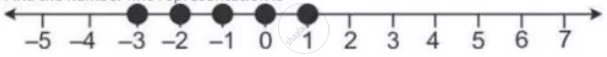Share

Books Shortlist

# Solve the Following Inequation and Represent the Solution Set on the Number Line 2x – 5 ≤ 5x +4 < 11, Where X ∈ I - ICSE Class 10 - Mathematics

ConceptRepresentation of Solution on the Number Line

#### Question

Solve the following inequation and represent the solution set on the number line 2x – 5 ≤ 5x +4 < 11, where x ∈ I

#### Solution

2x - 5 <= 5x + 4 and 5x + 4 < 11

2x - 5x <= - 5 and 5x < 11 - 4

3x <= -1 and 5x  < 7

x >= -1 and x < 1.4

Since x ∈ I, the solution set is {-3, -2 -1, 0, 1}

And the number line representation isIs there an error in this question or solution?

#### APPEARS IN

Solution Solve the Following Inequation and Represent the Solution Set on the Number Line 2x – 5 ≤ 5x +4 < 11, Where X ∈ I Concept: Representation of Solution on the Number Line.
S